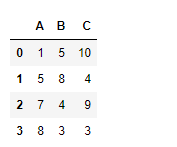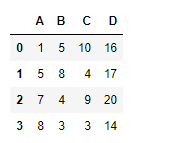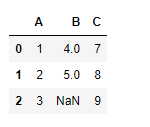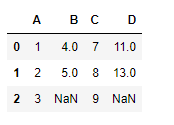GFG App
Open AppBrowser
Continue

# Python | Pandas dataframe.eval()

Python is a great language for doing data analysis, primarily because of the fantastic ecosystem of data-centric python packages. Pandas is one of those packages and makes importing and analyzing data much easier.

Pandas` dataframe.eval()` function is used to evaluate an expression in the context of the calling dataframe instance. The expression is evaluated over the columns of the dataframe.

Syntax: DataFrame.eval(expr, inplace=False, **kwargs)

Parameters:
expr : The expression string to evaluate.
inplace : If the expression contains an assignment, whether to perform the operation inplace and mutate the existing DataFrame. Otherwise, a new
DataFrame is returned.
kwargs : See the documentation for eval() for complete details on the keyword arguments accepted by query().

Returns: ret : ndarray, scalar, or pandas object

Example #1: Use `eval()` function to evaluate the sum of all column element in the dataframe and insert the resulting column in the dataframe.

 `# importing pandas as pd ` `import` `pandas as pd ` ` `  `# Creating the dataframe  ` `df``=``pd.DataFrame({``"A"``:[``1``,``5``,``7``,``8``], ` `                 ``"B"``:[``5``,``8``,``4``,``3``], ` `                 ``"C"``:[``10``,``4``,``9``,``3``]}) ` ` `  `# Print the first dataframe ` `df `Let’s evaluate the sum over all the columns and add the resultant column to the dataframe

 `# To evaluate the sum over all the columns ` `df.``eval``(``'D = A + B+C'``, inplace ``=` `True``) ` ` `  `# Print the modified dataframe ` `df `

Output :Example #2: Use `eval()` function to evaluate the sum of any two column element in the dataframe and insert the resulting column in the dataframe. The dataframe has `NaN` value.

Note : Any expression can not be evaluated over `NaN` values. So the corresponding cells will be `NaN` too.

 `# importing pandas as pd ` `import` `pandas as pd ` ` `  `# Creating the dataframe ` `df``=``pd.DataFrame({``"A"``:[``1``,``2``,``3``], ` `                 ``"B"``:[``4``,``5``,``None``], ` `                 ``"C"``:[``7``,``8``,``9``]}) ` ` `  `# Print the dataframe ` `df `Let’s evaluate the sum of column “B” and “C”.

 `# To evaluate the sum of two columns in the dataframe ` `df.``eval``(``'D = B + C'``, inplace ``=` `True``) ` ` `  `# Print the modified dataframe ` `df `

Output :Notice, the resulting column ‘D’ has `NaN` value in the last row as the corresponding cell used in evaluation was a `NaN` cell.

My Personal Notes arrow_drop_up